# An epidemic is a sequence of random events

If a contact is made, then whether or not infection is transferred is much like tossing a (loaded) coin. How can a simulation take all this uncertainty into account?

Bill Casselman
University of British Columbia

Just recently, I started thinking about making my own epidemics. On a computer, of course, with a digital population.

What I had in mind was not very sophisticated. I would assemble a population of people’, a few of which are infected with the virus, and then progress from one day to the next, letting the virus spread around through interactions in the population. At each moment, a person would be in one of several possible states:

• S Uninfected but susceptible to infection
• E Infected, but not yet infectious (exposed)
• A Infectious, but not yet showing symptoms (asymptomatic)
• Infectious and showing symptoms
• Recovered, or otherwise incapable of becoming infected (say, through vaccination)

This is not quite a complete representation of reality. For example, in the current epidemic a very small number of people get reinfected. But it is not too far from being correct.

In general, as the simulation goes on, a person would progress from one state in this list to the next, except of course that being vaccinated is a shortcut from the first to the last state. Infections take place because susceptible persons interact with contagious ones. Even when an interaction takes place, whether or not infection is transmitted is a function of many accidental circumstances (for example, surrounding ventilation) as well as how contagious the infected person is.

There is some further internal detail to some of these states. The degree to which a person is infectious changes in time, usually rising to a peak after a few days, and then decreasing to zero. Hence in a simulation each person has attached to him in addition to (i) a designation of state but also in states A and I (ii) a number measuring infectiousness. A further datum is (iii) the frequency of contacts, especially close contacts, a person has with others. This can change with time. For example, when a person starts showing symptoms, he will presumably reduce the frequency of his contacts.

Where’s the mathematics? An epidemic is driven by random events. The moment at which a person moves from one state to the next is not fixed by circumstances, but is instead a matter of probabilities. The severity of a person’s infection is a matter of chance, as is the length of time from when he is infected to when he becomes infectious. Even if we know the average rate at which an infectious person makes contacts, the exact number of contacts made in one day is also a matter of chance. If a contact is made, then whether or not infection is transferred is much like tossing a (loaded) coin. How can a simulation take all this uncertainty into account?

### Generating contacts

Take the matter of contacts. The most important parameter governing contacts is the average number $c$ of contacts made by a person in one day, but that does not mean that the number of contacts in one day is constant. It might well vary from day to day. Instead, it is reasonable to assume that personal interaction is a Poisson process, which means that the probability of making $k$ contacts during one day is $p_{k} = c^{k} e^{-c} / k!$. Note that the infinite sum of the $p_{k}$ is $1$, because of the well known formula

$$e^{c} = 1 + c + {c^{2} \over 2!} + { c^{3} \over 3! } + \cdots \, .$$

For example, here are the graphs of some examples with a couple of values of $c$: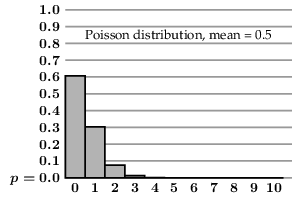In a simulation, one will be dealing with a large number of people. Each of them will have his own regimen of interactions. Some of them will be more interactive than others. Thus, we are likely to find ourselves simulating a large number of independent Poisson processes, each one a sequence of random events. How to do this? In a program, this will involve a call to a routine, call it p_random(c) that returns on each call a random non-negative integer whose distribution matches the Poisson process with mean $c$.

Almost every programming language has built into it a routine random() that does something like this. On each call it returns a real number uniformly distributed in the open interval $[0,1)$. (David Austin’s previous FC gives some idea of how this works.) What we would like to do is use that routine to generate non-negative integers following a specified Poisson distribution. To give you some idea of how things go, we can see how this technique can generate integers uniformly distributed in any integral range $[0,n-1]$: get a random number $x$ in $[0,1)$ and then replace it by $\lfloor nx \rfloor$, the integral part of $nx$. If $n=2$ this offers a simulation of coin tossing, and if $n=6$ a simulation of throwing a die.

There is a reasonably well known procedure that does what we want, and very generally. This is explained in Knuth’s classic text. Suppose we are given an arbitrary probability distribution of integers with given probabilities $p_{k}$ for $k \ge 0$. That is to say, we are looking at some repeated event somewhat like coin tossing, in which a non-negative integer $k$ occurs with probability $p_{k}$. How can a program generate integers distributed according to these statistics?

Let $P(k)$ be the cumulative distribution

$$P(k) = {\sum}_{i = 0}^{k} p(i)$$

Thus $P(k)$ is the probability that the integer $j$ occurring is $\le k$. The original distribution has the property that each $p_{i} \ge 0$ and ${\sum} p(i) = 1$, so $P(k)$ increases from $0$ to $1$. For example, if $c = 2.5$ and $p(k) = e^{-c} c^{k} / k!$ then the graph of $P$ looks like the figure below. Given a random number $t$ in $[0,1)$ we can determine an integer according to the recipe indicated—draw a line to the right from the point $(0,t)$ and select the $x$-coordinate of the point at which it hits this graph.There is another suggestive way to see this. Make up a rectangle of total height $1$, partitioned into boxes, with the one labeled $k$ of height $p_{k}$. Given the number $x$, mark a point at height $x$ in the rectangle. Select the label of the box that contains it.In the long run the number of times you hit the box labeled $k$ will be proportional to its area, hence to $p_{k}$. But how do you tell what that label is? There is one straightforward answer to this question:

def p_random():
x = random()
# this is the built-in random number generator
s = 0
i = 0
while s <= x:
i += 1
s += p[i]
# at exit p + ... + p[i-1] <= x < p[i]
return i-1


But this is somewhat inefficient, since each call will on average involve $n/2$ steps. Does there exist an algorithm that requires a number of steps independent of $n$? The answer is yes. A clever method whose basic idea is apparently due to Alastair Walker does this, at the small cost of building some preliminary structures.

## Walker’s trick

As far as I know, Walker never explained how he discovered his method, but an elegant interpretation has been offered by Keith Schwartz. The basic idea is what we have already seen:

1. Start with a box of some kind. Partition it into smaller labeled boxes in such a way that the area of box $k$ is proportional to $p_{k}$.
2. To generate integers with a given probability distribution, choose points at random inside the box, and return the label of the region hit.
3. Arrange a way to assign to every random $x$ in $[0,1)$ a point of the box.

The problem is to figure out how to make the partition in such a way that figuring out the label from the geometry of the partition can be done efficiently.

I’ll explain how Walker’s method works for a few simple cases, but first I’ll generalize the problem so that we are not restricted to the Poisson distribution. Suppose very generally that we are given probabilities $p_{i}$ for $i$ in $[0, n-1]$. We now want a method to generate random integers that follow the distribution assigned by $p_{i}$. That is to say, if we generate in this way a large number of integers, we want the proportion of occurrences of $i$ to be roughly $p_{i}$.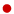The case $n=2$ is like tossing a biased coin, and there is a simple solution. In this case, we are given two probabilities $p_{0}$, $p_{1}$ with $p_{0} + p_{1} = 1$. Partition the unit square in this fashion: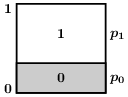Choose a point $(x, y)$ randomly in the square. In fact, we do not have to pay any attention to $x$. If $y \le p_{0}$ we return $i = 0$ and otherwise we return $i = 1$.But now, following Keith Schwartz and intending to show how Walker’s algorithm works in this very simple case, I will set up a rectangular region a bit differently. First of all, make its dimensions $2 \times 1$. Partition it twice: once into halves, each half a unit square …… and then build in each half, say in the $i$-th half, a box of dimensions $1 \times p_{i}$. Label these boxes. Unless $p_{0} = p_{1}$, one of these will overflow at the top: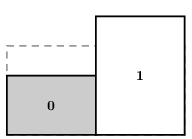So then we cut off the overflow and paste it (with label) into the other box: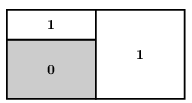This shows the case $p_{0} \le p_{1}$. If $p_{1} < p_{0}$ things look like this:How do we use these diagrams to generate the random integers we want? Choosing a random uniform number $x$ in $[0,1)$ amounts as before to choosing a point in the rectangle. But we do this differently, and we interpret it differently. Given $x$, set $X = 2x$. Let $m$ be the integer part of $X$, which will be either $0$ or $1$: $m = \lfloor X \rfloor$. Let $y = X – m$, the fractional part of $X$. Associate to $x$ a point in the $m$-th box with height $y$. If $y \lt p_{m}$, then we are in the box labeled by $m$, otherwise in the other one. In either case, the process will select that label $m$.Now look at the case $n = 3$, and suppose that we are given probabilities $p_{0}, p_{1}, p_{2}$ with $\sum p_{i} = 1$. We start off with a rectangle of size $3 \times 1$, partitioned into $1 \times 1$ boxes:There are again different cases, depending on the relative sizes of the $p_{i}$. The easiest case is that in which two of the values of $p$, say $p_{0}$ and $p_{1}$, are less than $1/3$, which implies that the third is greater than $1/3$. Draw the $i$ boxes of dimension $1 \times p_{i}$ in the $i$-th square, like this: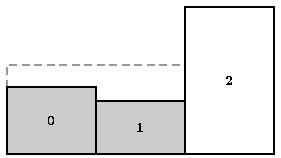Now cut off two pieces from the large box and paste them into the smaller one, getting: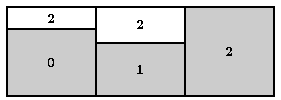I’ll explain in a moment how to use this to generate random numbers.

There is a second case, in which two of the $p_{i}$ are larger than $1/3$: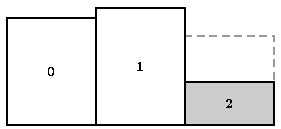Here, we want to cut off from the tops and fill in the third. It is tempting to cut off exactly the overflows in the large regions and paste them in, but this would give the third region three labels. which is not what we want. So we fill in from just one of the large regions. This will leave some space in it.We fill in the new empty space from the other large region. We are now finished: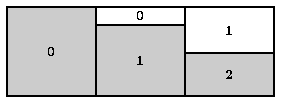How to use what we have constructed? In each case, we have partitioned the rectangle of size $3 \times 1$. First, into three unit squares, and then each of these in turn into one or two labeled rectangles. Given a random $x$ in $[0,1)$, we want to come up with some integer in $[0,3)$. How? We first scale it to get $X = 3x$. This will lie in the interval $[m, m+1)$ for $m = \lfloor X \rfloor$. We now turn attention to the $m$-th unit square. The integer we return will be one of the labels found in that square. Let $y = X – m$, the fractional part of $X$, which will be in $[0,1)$. If $y \lt p_{m}$ (the height of the bottom rectangle), p_random returns $m$, otherwise the alternate label in that square.

In effect we are assigning principal and alternate labels to the boxes. Except that there won’t be an alternate label if the box is full.

In the literature, the array I call alternate’ is called alias, and the method described here is called the alias method.

## The full algorithm

This method generalizes nicely. The original version seems to be due to Alastair Walker. It became well known because Knuth called attention to it (although mostly in exercises). Michael Vose then came up with a more efficient version, and made it handle rounding errors more stably.

I quote below, almost verbatim, the algorithm found originally in Vose’s paper. It improves the running time of Walker’s program, and corrects its handling of rounding errors. It has two parts. One is an initialization that sets up arrays prob and alias from the probability array $p$. These are used in the function rand, which returns a random variable in the range $[0,n-1]$, whose probabilities are specified in p of length n. The call to random returns a variable uniformly distributed in $[0, 1)$.

There are several loops in the program.The first assigns integers in the range $[0,n-1]$ to one of two explicit arrays large and small. Those in small are the $i$ such that $p_{i} \le 1/n$. As the program proceeds, the integers in these arrays are those whose boxes have not yet been filled. Implicit is a third subset of $[0,n-1]$, which I’ll call finished. This contains all those indices for which no further processing is necessary—i. e. whose box is filled.

In the loop  the two arrays small and large are initialized, and the subset finished is left empty. In every run through this loop, an index is taken from small, its square is filled, and it is added to FIN. This happens by removing filling material form one of the boxes in large, which therefore becomes smaller. It is added to either small or large, according to how much is left. In each of these loops, the total size of large and small is decremented.

def init(p):
l = 0
s = 0
 for i in range(n):
if p[i] > 1/n:
large[l] = i
l += 1
else:
small[s] = i
s += 1
 while s > 0 and l > 0:
s -= 1
j = small[s]
l -= 1
k = large[l]
prob[j] = n*p[j]
alias[j] = k
p[k] += (p[j]-b)
if p[k] > b:
large[l] = k
l += 1
else:
small[s] = k
s += 1
 while s > 0:
s -= 1
prob[small[s]] = 1
 while l > 0:
l -= 1
prob[large[l]] = 1

def p_random():
x = n*random(0, 1)
m = floor(x)
if (x - m) < prob[m]: return m
else: return alias[m]


The last loops  and  of Vose’s code are necessary to deal with rounding errors, which I include without further comment.

Here is a typical run for a Poisson process with mean $\mu = 4$.## The simulation

Let’s see how to use Walker’s method to simulate how a person just infected goes on to infect others. Suppose that he starts to be infectious on the fifth day, and that the probability that he infects a contact is specified in the following table:

$$\matrix { i = \hbox{day after infection} & 1 & 2 & 3 & 4 & 5 & 6 & 7 & 8 & 9 & \ge 10 \cr r_{i} = \hbox{probability of infection} & 0 & 0 & 0 & 0 & 0.1 & 0.3 & 0.4 & 0.4 & 0.2 & 0 \cr }$$

Suppose also that he makes and average of $4$ close contacts per day, and that these follow a Poisson distribution. Applying Walker’s algorithm, we get a sample run of contacts like this:

$$\matrix { i = \hbox{day after infection} & 1 & 2 & 3 & 4 & 5 & 6 & 7 & 8 & 9 & 10\ \dots \cr c_{i} = \hbox{number of contacts} & 5 & 2 & 3 & 4 & 3 & 3 & 2 & 1 & 3 & 3\ \dots \cr }$$

In this run, how many people does he infect? There is no unique answer to this question. It depends on something like a coin toss at each contact. What we can calculate is an average. On the fifth day he infects an average of $0.1 \cdot 3$ people, on the sixth … etc. All in all:

$$\hbox{average number of infections} = {\sum} c_{i} r_{i} = 0.1 \cdot 3 + 0.3 \cdot 3 + 0.4 \cdot 2 + 0.4 \cdot 1 + 0.2 \cdot 3 = 3.0 \, .$$

This is much lower than the average number of people he infects, which is called the $R_{0}$ value for this example. Here it is $(0.1 + 0.3 + 0.4 + 0.4 + 0.2) \cdot 4 = 5.6$.

• Keith Schwartz’ discussion of the alias algorithm as dart throwing.I have taken the graphical interpretation of Walker’s algorithm from this exhaustive treatment.
• Alastair Walker, An efficient method for generating discrete random variables with general distributions, ACM Transactions on Mathematical Software 3, 1977.
• Michael Vose, A linear algorithm for generating random numbers with a given distribution, IEEE Transactions on Software Engineering 17, 1991.
• Donald Knuth, The art of computer programming, Volume II on seminumerical algorithms.Section 3.4 deals with the problem of generating random numbers with a given probability distribution.
• David Austin, Nothing left to chance.An explanation of how a computer generates uniformly distributed (pseudo-)random numbers.
• Python code for init and p_random.

### 1 Comment

1.Interesting post. I didn’t know about this clever algorithm for generating random variables from a given discrete distribution. However, there are two small errors in the above post.
First, in your analysis of the basic algorithm you say that it involves “on average n/2 steps.” Assuming (as you did with the algorithm) that the random variable has values 1,2,…,n, then if the algorithm returns the value i it will have run for i+1 loops. Thus, the average run time will be $mu$+1 where $mu$ is the the expected value of the distribution in question.
A second minor quibble is that the algorithms you discuss are only for finite probability distributions, but then you discuss applying them to the Poisson distribution without mentioning that of course you must really be generating a truncated Poisson distribution (and one must make the choice of where to truncate the distribution).